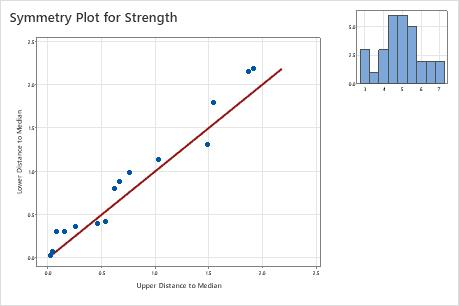# Overview for Symmetry Plot

Use Symmetry Plot to assess whether sample data come from a symmetric distribution. Some statistical procedures require that data come from a normal distribution, but many procedures are robust to violations of normality. Thus, having data from a symmetric distribution is often adequate.

For example, an engineer wants to know whether plastic strength data is symmetric. The plot shows that the data appear to be symmetrically distributed, with no major deviations.## Where to find this analysis

To create a symmetry plot, choose Stat > Quality Tools > Symmetry Plot.

## When to use an alternate analysis

If you need to know whether you data come from a normal distribution, use Normality Test.

By using this site you agree to the use of cookies for analytics and personalized content.  Read our policy